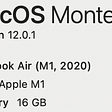# A dashboard to visualize DeGroot learning on networks with Python and Streamlit

DeGroot shows how opinions are formed and consensus arises when people or entities take in account others’ opinions to update their own. It also gives an intuition about the meaning of “eigenvector centrality” as a measure of influence. Sources: https://github.com/antigones/streamlit-degroot

In the DeGroot model, people have opinions and those opinions are represented with a vector of probabilities, p. Probabilities can represent some kind of belief about a subject, the likeliness of an event to occur, how much the agent likes something. Society is modeled with a stochastic matrix, representing connections and interaction between individuals: value Tij is the weight agent i gives to j’s opinion when it comes to update i’s own.

As an example, take the following matrix:

In this society, agent 1 weights own belief as 2 but does not care about agent 3; agent 2 weights own belief as much as 3 but does not care about 1; agent 3 only cares about agent 1.

Suppose p(0) to be as following:

We can think that this specific vector represents the fact that agent 1 fully believes that an event will occur, while agents 2 and 3 are totally skeptical about this event to take place.

At every step beliefs get updated in the following way:

Updating the vector is simulating people taking in account other peoples’ opinions and updating their own at any given time.

Applying some math and solving p(t) equality, it is possible to observe that:

and to realize that, on the long term, the result of the updating process is more about T^t than about p(0)

In fact, when the limit exists, beliefs converge and agents reach a consensus:

and in this setting, agent 1 and agent 2 have far more influence than agent 1:

Note also that

(2/5, 2/5, 1/5)

is also an eigenvector for T: DeGroot also gives a glimpse about the meaning of eigenvector centrality as a measure of social influence.

# Developing a dashboard to visualize DeGroot convergence

To visualize how DeGroot evolves over time over a sample society, using Python turned out to be a fairly good choice (numpy is awesome to perform matrix math!).

We can define deGroot as a function of the society (environment) and initial beliefs vector (beliefs). Keeping track of consensus reaching and a variable “i” to stop the algorithm when it does not converge (1000 is a symbolic upper limit), we update the beliefs vector by multiplying T by p.

Check for convergence is done by inspecting the difference between previous beliefs vector and current one: if it is sufficiently small then we assume that the convergence has been reached and exit the loop.

import numpy as npdef deGroot(environment, beliefs):    i = 0    consensus = False    while (consensus == False and i < 1000):        old_beliefs = beliefs        beliefs = environment @ beliefs        if (np.all(old_beliefs — beliefs < np.full(len(beliefs),sys.float_info.epsilon))):            consensus = True        i = i + 1    return beliefs

To track the consensus reaching steps, we define a similar function:

def deGrootSteps(environment, beliefs):    steps = []    i = 0    consensus = False    while (consensus == False and i < 1000):old_beliefs = beliefs        beliefs = environment @ beliefs        if (np.all(old_beliefs — beliefs < np.full(len(beliefs),sys.float_info.epsilon))):            consensus = True         i = i + 1         steps.append(beliefs)     return steps

which basically fill an array of “steps” to consensus.

We also add an helper function to format decimal as fraction (we don’t want to visualize matrices with very long numbers) and a function to display matrix as Latex formula:

from fractions import Fraction as fracimport numpy as npdef convert_to_frac(mat):    result = []    mat_list = mat.astype(str).tolist()    for i in range(0, len(mat_list)):        result.append([])        for j in range(0, len(mat_list[i])):            result[i].append(            str(frac(str(mat_list[i][j])).limit_denominator(10)))    return resultdef convert_to_latex(mat):    mat_list = convert_to_frac(mat)    mat_list_rows = list(map(r” & “.join, mat_list))    mat_latex_content = r” \\\ “.join(mat_list_rows)    return mat_latex_content

Streamlit is a quite nice package to easily create interactive web apps with python.

Just add it to the virtual environment by typing:

pip install streamlit

Streamlit provides nice components to interact with (slider, texts, latex formulae…).

We add a title and some descriptive text:

import streamlit as stst.title("DeGroot")st.write("DeGroot gives an insight about how network topology influence spread of information and opinion formation.It also gives an intuition about the reason why eigenvector centrality expresses influence.")st.write("Move the slide to inspect the belief vector as it updates according to DeGroot model.")

We also want to add a slider to control the simulation:

p0 = np.matrix([1, 0, 0]).TT = np.matrix([[1/2, 1/2, 0], [0, 1/2, 1/2], [1, 0, 0]])dg_steps = dg.deGrootSteps(T, p0)step = st.slider('Step', 0, len(dg_steps)-1, 0)st.write("Step: ", step, '')

We can also begin to inspect how the app will look like by launching our py script as a streamlit “app”:

streamlit run app.py

The browser will open the app page:

Note that the number of steps will update accordingly to slider value.

We can use networkx to draw network topology and Streamlit’s “graphviz_chart”:

G = nx.from_numpy_matrix(T, create_using=nx.MultiDiGraph())…T_label = u.convert_to_frac(T)for i, j, d in G.edges(data=True):    d[‘label’] = T_label[i][j]dot = nx.nx_pydot.to_pydot(G)col1, col2 = st.beta_columns(2)with col1:    st.graphviz_chart(dot.to_string())

As Streamlit can display Latex, we use st.latex to display society matrix and beliefs vector:

ltx_mat = u.convert_to_latex(T)b_mat = u.convert_to_latex(dg_steps[step])p0_mat = u.convert_to_latex(p0)col3, col4, col5 = st.beta_columns(3)with col3:    st.latex(r'''    T =    \begin{pmatrix}    '''+ltx_mat+r'''    \end{pmatrix}    ''')with col4:    st.latex(r'''    p(0) =    \begin{pmatrix} ''' + p0_mat + r'''\end{pmatrix}    ''')with col5:    st.latex(r'''    T^{t}p(0) {\rightarrow}    \begin{pmatrix}    ''' + b_mat + r'''    \end{pmatrix}    ''')

We also display Ttconvergence in the same way (with some index adjustment to skip step 0):

st.title("Convergence of T")T_t = np.linalg.matrix_power(T, step+1)T_t_mat = u.convert_to_latex(T_t)st.latex(r'''    T^{t} {\rightarrow}    \begin{pmatrix}    ''' + T_t_mat + r'''    \end{pmatrix}    ''')

And here’s the final result:

References: “Social and economic networks”, Mattew O. Jackson, Princeton; Wikipedia, https://en.wikipedia.org/wiki/DeGroot_learning

--

--

--

## More from Juna Salviati

Full-time Human-computer interpreter. Opinions are my own.

Love podcasts or audiobooks? Learn on the go with our new app.

## Recommended from Medium## NeuroNuggets: ACL in Review I## Building Domain Specific Language Models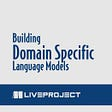## Introducing Prefect-ML: Orchestrate a Distributed Hyperparameter Grid Search on Dask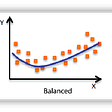## Hyperparameter Tuning — Always Tune your Models## Machine Learning — where to start ?## Monday Notes 8–27–18## Why care about structuring a deep learning project ?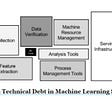## Juna Salviati

Full-time Human-computer interpreter. Opinions are my own.

## Telemetry Dashboard for AI Applications using Plotly Dash## EDA of Kaggle Image Data Set by Using Plotly and Pandas Data Frame!## My first Streamlit app using choropleth map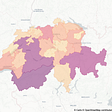## Set up python environment (with TensorFlow) on M1 Mac